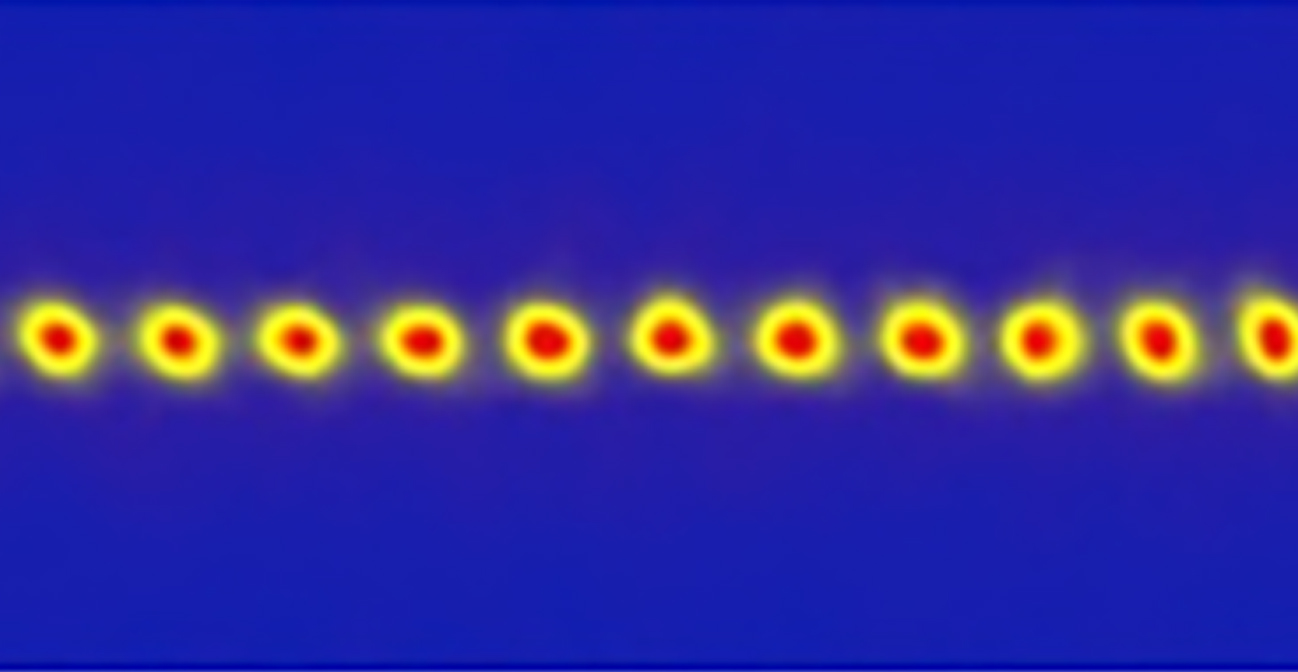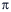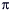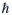# The Quantum WorldThis unit covers a field of physics that is simultaneously one of the most powerful, transformational, and precise tools for exploring nature and yet for non-physicists one of the most mysterious and misunderstood aspects of all science. Developed early in the 20th century to solve a crisis in understanding the nature of the atom, quantum mechanics has laid the foundation for theoretical and practical advances in 21st century physics. The unit details the reasoning that led to ever deeper awareness of the nature of particles, waves, and their interrelationships, provides a primer on present-day understanding of the field, and outlines ways in which that understanding has led to significant applications today.

### Unit Glossary

alkali metals

The alkali metals are the chemical elements in the first column of the periodic table. They all have one valence electron. Alkali metals are commonly used atoms in atomic physics experiments for several reasons. Their structure is relatively simple and provides energy states that are convenient for laser cooling. Many of their transition frequencies match convenient laser sources. Also, the single valence electron’s magnetic moment allows the atoms to be easily trapped using magnetic fields, which is convenient for the evaporative cooling process necessary to reach ultracold temperatures.

atomic fountain

An atomic fountain is a cloud of cold atoms that is given a push upward with a laser pulse. The laser is tuned to the right energy to transfer its momentum to the atoms, which fly up until gravity takes over, reversing their motion so they fall back down. The path the atoms take is analogous to the path of water in a fountain.

blackbody

A blackbody is an object that absorbs all incident electromagnetic radiation and re-radiates it after reaching thermal equilibrium. The spectrum of light emitted by a blackbody is smooth and continuous, and depends on the blackbody’s temperature. The peak of the spectrum is higher and at a shorter wavelength as the temperature increases.

Bohr Correspondence Principle

The Bohr Correspondence Principle states that the predictions of quantum mechanics must match the predictions of classical physics in the physical situations that classical physics is intended to describe, and does describe very accurately. Mathematically, this means that the equations of quantum mechanics must smoothly turn into the equations of classical mechanics as the de Broglie wavelength of particles becomes very small, and the energy state quantum number gets very large.

Bose-Einstein condensate

A Bose-Einstein condensate, or BEC, is a special phase of matter in which the quantum mechanical wavefunctions of a collection of particles line up and overlap in a manner that allows the particles to act as a single quantum object. The electrons in a superconductor form a BEC; superfluid helium is an example of a liquid BEC. BECs can also be created from dilute gases of ultracold atoms and molecules.

cosmic microwave background

The cosmic microwave background (CMB) radiation is electromagnetic radiation left over from when atoms first formed in the early universe, according to our standard model of cosmology. Prior to that time, photons and the fundamental building blocks of matter formed a hot, dense soup, constantly interacting with one another. As the universe expanded and cooled, protons and neutrons formed atomic nuclei, which then combined with electrons to form neutral atoms. At this point, the photons effectively stopped interacting with them. These photons, which have stretched as the universe expanded, form the CMB. First observed by Penzias and Wilson in 1965, the CMB remains the focus of increasingly precise observations intended to provide insight into the composition and evolution of the universe.

diffraction

Diffraction is the spreading of a wave after it encounters an obstacle, a sharp corner, or emerges from a slit. If the slit is small, the spreading is large and is accompanied by an interference pattern with a central peak surrounded by weaker side lobes. In this context, “small” means comparable to the wavelength of the diffracting wave. The fact that light diffracts when passed through a small slit is evidence of its wave nature.

Doppler cooling

Doppler cooling is a technique that uses laser light to slow, and thus cool, moving atoms. An atom will absorb a photon that has an energy equal to the difference between two energy levels in the atom. When the atom absorbs a photon, it also absorbs the photon’s momentum and gets a push in the direction that the photon was traveling. If the photon and atoms were traveling in opposite directions, the atom slows down. However, when the atom is moving relative to the laser, the laser light is Doppler shifted in the atom’s reference frame. To cool moving atoms, the laser must be tuned slightly to the red to account for the Doppler shift of atoms moving toward the light source.

Doppler shift (Doppler effect)

The Doppler shift is a shift in the wavelength of light or sound that depends on the relative motion of the source and the observer. A familiar example of a Doppler shift is the apparent change in pitch of an ambulance siren as it passes a stationary observer. When the ambulance is moving toward the observer, the observer hears a higher pitch because the wavelength of the sound waves is shortened. As the ambulance moves away from the observer, the wavelength is lengthened and the observer hears a lower pitch. Likewise, the wavelength of light emitted by an object moving toward an observer is shortened, and the observer will see a shift to blue. If the light-emitting object is moving away from the observer, the light will have a longer wavelength and the observer will see a shift to red. By observing this shift to red or blue, astronomers can determine the velocity of distant stars and galaxies relative to the Earth. Atoms moving relative to a laser also experience a Doppler shift, which must be taken into account in atomic physics experiments that make use of laser cooling and trapping.

evaporative cooling

Evaporative cooling is a process used in atomic physics experiments to cool atoms down to a few billionths of a degree above absolute zero. The way it works is similar to how a cup of hot coffee cools through evaporation. Atoms are pre-cooled, usually with some kind of laser cooling, and trapped in a manner that imparts no additional energy to the atoms. The warmest atoms are removed from the trap, and the remaining atoms reach a new, lower equilibrium temperature. This process is typically repeated many times, creating small clouds of very cold atoms.

frequency comb

A frequency comb is a special type of laser that has a spectrum that looks like a comb. Most lasers emit light at one well-defined frequency, and have a resonance curve that looks like a peak at that frequency and zero everywhere else. A frequency comb has a resonance curve that looks like a series of evenly spaced peaks over a broad range. These lasers were important in the development of optical clocks because the peaks in their spectrum are at optical frequencies, but the spacing between peaks is at much lower microwave frequencies. Comb lasers therefore provide a link between optical frequencies that are very difficult to measure directly, and microwave frequencies that can be counted with well-established laboratory techniques.

ground state

The ground state of a physical system is the lowest energy state it can occupy. For example, a hydrogen atom is in its ground state when its electron occupies the lowest available energy level.

harmonic oscillator

A harmonic oscillator is a physical system that, when displaced from equilibrium, experiences a restoring force proportional to the displacement. A harmonic oscillator that is displaced and then let go will oscillate sinusoidally. Examples from classical physics are a mass attached to a spring and a simple pendulum swinging through a small angle.

Heisenberg uncertainty principle

The Heisenberg uncertainty principle states that the values of certain pairs of observable quantities cannot be known with arbitrary precision. The most well-known variant states that the uncertainty in a particle’s momentum multiplied by the uncertainty in a particle’s position must be greater than or equal to Planck’s constant divided by 4. This means that if you measure a particle’s position to better than Planck’s constant divided by 4, you know that there is a larger uncertainty in the particle’s momentum. Energy and time are connected by the uncertainty principle in the same way as position and momentum. The uncertainty principle is responsible for numerous physical phenomena, including the size of atoms, the natural linewidth of transitions in atoms, and the amount of time virtual particles can last.

hyperfine interaction

When the nucleus of an atom has a non-zero magnetic moment, the magnetic field of the nucleus interacts with electrons in the atom. This interaction is called the hyperfine interaction, and leads to finely spaced atomic energy levels called hyperfine structure.

interference

Interference is an effect that occurs when two or more waves overlap. In general, the individual waves do not affect one another, and the total wave amplitude at any point in space is simply the sum of the amplitudes of the individual waves at that point. In some places, the two waves may add together, and in other places they may cancel each other out, creating an interference pattern that may look quite different than either of the original waves. Quantum mechanical wavefunctions can interfere, creating interference patterns that can only be observed in their corresponding probability distributions.

magnetic moment

The magnetic moment (or magnetic dipole moment) of an object is a measure of the object’s tendency to align with a magnetic field. It is a vector quantity, with the positive direction defined by the way the object responds to a magnetic field: The object will tend to align itself so that its magnetic moment vector is parallel to the magnetic field lines. There are two sources for a magnetic moment: the motion of electric charge and spin angular momentum. For example, a loop of wire with a current running through it will have a magnetic moment proportional to the current and area of the loop, pointing in the direction of your right thumb if your fingers are curling in the direction of the current. Alternatively, an electron, which is a spin-1/2 fermion, has an intrinsic magnetic moment proportional to its spin.

magneto-optical trap

A magneto-optical trap, or MOT, uses a combination of laser beams and magnetic fields to confine atoms at temperatures between a few millikelvin and a few microkelvin. Atoms in a MOT are constantly interacting with the laser beams, which cool them to the laser-cooling limit, but no further than that.

matrix mechanics

Matrix mechanics is the version of quantum mechanics formulated in the 1920s by Werner Heisenberg and his close colleagues Max Born and Pascual Jordan. It makes extensive use of matrices and linear algebra, which was relatively new mathematics at the time. Matrix mechanics, which is mathematically equivalent to Schrödinger’s wave mechanics, greatly simplifies certain calculations.

microkelvin

The microkelvin is a unit of temperature equivalent to one-millionth (10-6) of a degree in the Kelvin scale. Laser-cooled atoms are typically at temperatures of a few microkelvin.

millikelvin

The millikelvin is a unit of temperature equivalent to one-thousandth (10-3) of a degree in the Kelvin scale. 3He becomes a superfluid at a temperature of around one millikelvin.

natural linewidth

The natural linewidth of an atomic energy level is the intrinsic uncertainty in its energy due to the uncertainty principle.

optical dipole trap

An optical dipole trap is a type of atom trap that uses only laser light to trap atoms. The laser frequency is tuned to a frequency below an atomic resonance so the atoms do not absorb laser photons as they do in laser cooling or in a MOT. Instead, the electric field from the laser induces an electric dipole in the atoms that attracts them to regions of more intense laser light. Optical dipole traps are only strong enough to hold cold atoms, so atoms are typically cooled first and then transferred into the dipole trap.

optical lattice

An optical lattice is an optical dipole trap made from a standing wave laser beam, so there is a periodic array of regions with a strong and weak laser field. Atoms are attracted to regions of a strong field, so they are trapped in a lattice-like pattern.

optical molasses

Optical molasses is formed when laser beams for Doppler cooling are directed along each spatial axis so that atoms are laser cooled in every direction. Atoms can reach microkelvin temperatures in optical molasses. However, the molasses is not a trap, so the atoms can still, for example, fall under the influence of gravity.

phase

In physics, the term phase has two distinct meanings. The first is a property of waves. If we think of a wave as having peaks and valleys with a zero-crossing between them, the phase of the wave is defined as the distance between the first zero-crossing and the point in space defined as the origin. Two waves with the same frequency are “in phase” if they have the same phase and therefore line up everywhere. Waves with the same frequency but different phases are “out of phase.” The term phase also refers to states of matter. For example, water can exist in liquid, solid, and gas phases. In each phase, the water molecules interact differently, and the aggregate of many molecules has distinct physical properties. Condensed matter systems can have interesting and exotic phases, such as superfluid, superconducting, and quantum critical phases. Quantum fields such as the Higgs field can also exist in different phases.

photon

Photons can be thought of as particle-like carriers of electromagnetic energy, or as particles of light. In the Standard Model, the photon is the force-carrier of the electromagnetic force. Photons are massless bosons with integer spin, and travel through free space at the speed of light. Like material particles, photons possess energy and momentum.

Planck’s constant

Planck’s constant, denoted by the symbol h, has the value 6.626 x 10-34 m2 kg/s. It sets the characteristic scale of quantum mechanics. For example, energy is quantized in units of h multiplied by a particle’s characteristic frequency, and spin is quantized in units of h/2. The quantity h/2appears so frequently in quantum mechanics that it has its own symbol:.

plane wave

A plane wave is a wave of constant frequency and amplitude with wavefronts that are an infinitely long straight line. Plane waves travel in the direction perpendicular to the wavefronts. Although they are a mathematical abstraction, many physical waves approximate plane waves far from their source.

polarization

The polarization of a wave is the direction in which it is oscillating. The simplest type of polarization is linear, transverse polarization. Linear means that the wave oscillation is confined along a single axis, and transverse means that the wave is oscillating in a direction perpendicular to its direction of travel. Laser light is most commonly a wave with linear, transverse polarization. If the laser beam travels along the x-axis, its electric field will oscillate either in the y-direction or in the z-direction. Gravitational waves also have transverse polarization, but have a more complicated oscillation pattern than laser light.

probability distribution

In quantum mechanics, the probability distribution is a mathematical function that gives the probability of finding a particle in any small region of space. The probability distribution for a quantum mechanical system is simply the square of the wavefunction.

quantized

Any quantum system in which a physical property can take on only discrete values is said to be quantized. For instance, the energy of a confined particle is quantized. This is in contrast to a situation in which the energy can vary continuously, which is the case for a free particle.

quantum number

A quantum number is a number that characterizes a particular property of a quantum mechanical state. For example, each atomic energy level is assigned a set of integers that is uniquely related to the quantized energy of that level.

resonance curve

A resonance curve is a graph of the response of an atomic system to electromagnetic radiation as a function of the frequency of the radiation. The simplest example of a resonance curve is the single peak that appears as a laser’s frequency is scanned through the difference between two energy levels in the atoms.

spontaneous emission

An atom in an excited state can decay down to a lower state by emitting a photon with an energy equal to the difference between the initial, higher energy level and the final, lower energy level. When this process takes place naturally, rather than being initiated by disturbing the atom somehow, it is called spontaneous emission.

standing wave

A standing wave is a wave that does not travel or propagate: The troughs and crests of the wave are always in the same place. A familiar example of a standing wave is the motion of a plucked guitar string.

stationary states

In quantum mechanics, a stationary state is a state of a system that will always yield the same result when observed in an experiment. The allowed energy states of a harmonic oscillator (Unit 5, section 5) are an example, as are the allowed energy levels of an atom. Stationary states correspond to quantum wavefunctions that describe standing waves.

superposition principle

Both quantum and classical waves obey the superposition principle, which states that when two waves overlap, the resulting wave is the sum of the two individual waves.

tunneling

Tunneling, or quantum tunneling, takes place when a particle travels through a region that would be forbidden according to the laws of classical physics. Tunneling occurs because quantum wavefunctions extend slightly past the boundaries that define where a particle is allowed to be. For example, in classical physics, an electron is allowed to move through a conductor but not through an insulator. However, if a thin layer of insulator is placed between two conductors, the electron can tunnel through from one conductor to the other because its wavefunction extends into the insulating layer.

wave mechanics

Wave mechanics is the version of quantum mechanics formulated primarily by Erwin Schrödinger in the 1920s. Following de Broglie’s hypothesis that particles can equally well be described as waves, Schrödinger set out to write down a wave equation for quantum systems and proceeded to solve it in many interesting examples. Wave mechanics is mathematically equivalent to Heisenberg’s matrix mechanics.

Zeeman effect

Each atomic energy level in which an atom has a non-zero spin splits into two or more separate levels when the atom is placed in an external magnetic field. The splitting grows with the strength of the external field. This effect is named the Zeeman effect after the experimentalist who first studied it in the laboratory, Pieter Zeeman. He received the 1902 Nobel Prize for this work, along with Hendrik Lorentz, the theorist who explained the effect.

zero point energy

The zero point energy is the minimum energy a system can have based on the Heisenberg uncertainty principle.

### Content Developer: Daniel KleppnerDaniel Kleppner is the Lester Wolfe Professor of Physics, Emeritus at MIT. He is the founding director of the MIT-Harvard Center for Ultracold Atoms, funded by the National Science Foundation. He is the co-author of two textbooks and the recipient of the Oersted Medal of the National Association of Physics Teachers and of the National Medal of Science.

### Featured Scientist: David J. WinelandDavid J. Wineland received a bachelor’s degree from Berkeley in 1965 and his Ph.D. from Harvard in 1970. After a postdoctoral appointment at the University of Washington he joined the National Bureau of Standards, presently renamed the National Institute of Standards and Technology (NIST), at Boulder, Colorado, where he is the leader of the Ion-Storage Group in the Time and Frequency Division. Dr. Wineland and Hans Dehmelt were the first to propose using lasers to cool trapped atomic ions, and, in 1978 he and coworkers conducted experiments that realized this effect. This early work led to a number of experiments in laser cooling and spectroscopy of trapped atomic ions, atomic clocks, quantum information processing, quantum-limited metrology, and quantum state control.